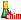Search:

# The molecular orbitals

A molecule is a much more complicated structure than a single atom. Physicists have proven that molecular orbitals can be foreseen using atomic orbitals:Two $1s$ orbitals (for instance from two separated hydrogen atoms) contain each one electron (symbolized by an arrow). Forming a bond (for instance $H-H$), these two orbitals "merge head on". The result is a new orbital of a new molecule. This molecular orbital is called $\sigma$ (sigma) and contains the two electrons.Two $2p_y$ orbitals sharing the same axis (f.i. in two separated oxygen atoms) contain each one electron (symbolized by an arrow). Forming the $O-O$ bond, these two orbitals "merge" head on . The result is a new orbital of a new molecule. This orbital is still called $\sigma$ (sigma)and contains the two former electrons . Attention! The schematic drawing above contains only one $\sigma$ orbital!Two $2p_z$ orbitals with parallel axes (f.i. in two separated oxygen atoms) contain each one electron (symbolized by an arrow). Forming another $O-O$ bond, these two orbitals "merge" laterally . The result is a new orbital of a new molecule. This orbital is called $\pi$ (pi) and contains the two former electrons. Attention! The schematic drawing above contains only one $\pi$ orbital!